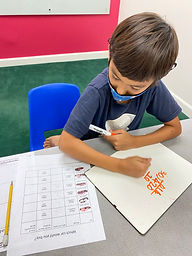## Ms. Kylie

### Target 1​

###### Lesson Type:

New

Data Probability

:

Ratio

Use ratio understandings to identify a unit rate.

###### 1:

Describe a unit rate in the form a:b and a/b.

###### 2:

Calculate unit rates.

###### 3:

Understand that a fraction, decimal, and percentage are all different forms of a ratio.

6th

###### Vocabulary:

Unit Rate, Rate, Ratio

Activities:

-Students were given word problems with ratios and solved the problems to find the unit rate.

-Students completed an activity where they compared different cars based on unit rate of miles per gallon and the cost of car insurance per month. Then they selected the car that they would like to buy based on the information for gas and insurance.### Home Exploration

###### Guiding Questions:## Absent Students:

### Target 2

:

###### 1:

Use the given information in a problem to identify the correct answer.

###### 2:

Use the given information to determine which is not the correct answer.

###### 3:

Think outside of the box to solve problems.

###### 4:

Follow given rules to solve problems.

###### Vocabulary:

Equivalent Sum

Activities:

-Students solved magic square puzzles. A magic square is made up of numbers arranged so that the numbers in each row and column equal to an equivalent sum. The sum is called the "magic number."

-Students used the given numbers to find the missing numbers for each puzzle. They used addition and subtraction to find the missing the numbers.### Home Exploration

###### Guiding Questions:### Target 3

:

###### Vocabulary:

Activities:### Home Exploration